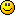#### Topic: Modifying iTexture or IImage per pixel

Hi,

I've trying to draw directly to screen for drawing antialiased 2d lines, the best way looks like directly drawing to a iTexture and the using MyDriver.Draw2dImage()... to get it on screen, however i've tried the code below but it looks like it must be messing with the memory directly because i see it drawing other textures, switching between random textures and colors? (its messy code atm because i was try multiple things, Sorry). I don't realy have anywhere to upload the example pictures but i could email if you wanted to see the issues when using setpixel()?

``````    Local Itex:IImage = Mydriver.createImageEmpty(3, _DIMENSION2DI(256, 256))
Local Col:SColor = _SCOLOR(255, 255, 255, 255)

Local Vect1:Vector2di = Vector2di.createFromVals(Startpos.getX(), Startpos.getY())
Local Vect2:Vector2di = Vector2di.createFromVals(EndPos.getX(), EndPos.getY())

Local Pos:Position2di = StartPos
Local Ang:Double = Vect1.getAngleWith(Vect2)
Local Dist:Double = Vect1.getDistanceFrom(Vect2)

Local SinVal:Double = Sin(Ang)
Local CosVal:Double = Cos(Ang)

Local A:Int
While A < Dist
A = A + 1
Itex.setPixel(Pos.getX(), Pos.getY(), Col, False)
'
Pos = _POSITION2DI(Pos.getX() + (SinVal * A), Pos.getY() + (CosVal * A))
Wend

'same as Driver.Draw2dImage() but just includes alpha by default
MyGUI.DrawFull2DImage(Texy, _POSITION2DI(50, 50))

MyDriver.removeTexture(Texy)
Itex.drop()``````

otherwise i've found this code which i think would be better anyway, but i don't know to use the texture.lock() function and how to access the data it provides through the byte ptr? if i could get help converting this for bmax that would be awesome``````video::ITexture* t = mesh->getMesh(0)->getMeshBuffer(0)->getMaterial().getTexture(0);
video::SColor c = video::SColor(0xFFFF0000);
int p = t->getPitch();
video::ECOLOR_FORMAT f = t->getColorFormat();
int z = video::IImage::getBitsPerPixelFromFormat(f) / 8;
int m = 0; // can be from 0 to 8 (for texture with size 256x256)
void* b = t->lock(false, m);
if (b)
{
for (int x = 0; x < (256 >> m); x++)
for (int y = 0; y < (256 >> m); y++)
c.getData((unsigned char*)b + y * (p / (1 << m)) + x * z, f);

t->unlock();
}``````